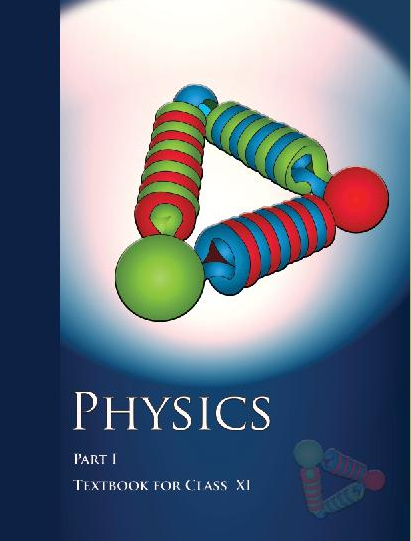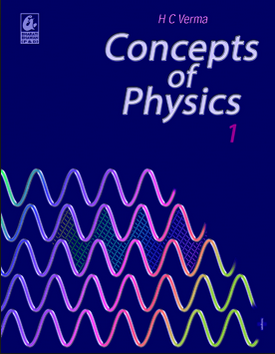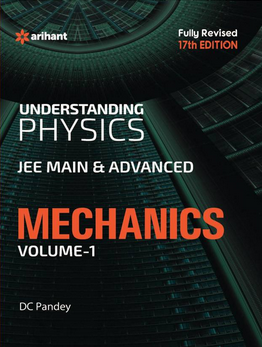# Physics and Measurement (Weightage 4%)   Share

## How to prepare Physics and Measurement for JEE Main 2020 2020

Most of the previous year's questions from this chapter are from dimensional formulae and dimensional analysis. This questions can be solved easily if the physics course is completed. No need for memorizing all dimensional formula. Dimensional formulae can be derived from basic equations easily. For example

Question- Dimensions of, where symbols have their usual meaning, are (AIEEE 2003)

 a) b) c) d)

This can be solved using:

The relation speed of light Therefor dimension of a  = dimension of =

• Another important section in this chapter is Errors In Measurement. This concept is used in the chapter Experimental Skills and in many problems from other chapters.

For Example- calculation of absolute error for a set of readings of the period of a simple pendulum etc.

Let's see another example from Errors

Question- The percentage of errors in quantities P, Q, R, and S are 0.5%, 1%, 3%, and 1.5%

respectively in the measurement of a physical quantity
(JEE Main 2018)

The maximum percentage error in the value of A will be :

This can be solved using the concept :

Error in quantity raised to some power -- wherein= absolute error in the measurement of a= absolute error in the measurement of b= absolute error in the measurement of x

=

• Significant figures is another topic from where the question is expected

For Example:

Question- The respective number of significant figures for the numbers 23.023, 0.0003 and 2.1 x 10-3 are

 a) 4, 4, 2 b) 5, 1, 2 c) 5, 1, 5 d) 5, 5, 2

Solution:

1)    23.023 has 5 significant digits (Any zeros between two significant digits are significant)

2)     0.0003 has only 1 significant digit as zero to the left of first non zero digits are not significant.

3) 2.1 x 10-3  It has two significant digits (A final zero or trailing zeros in the decimal portion ONLY are significant).

## TIPS

• Maximum Error in

1. Product x=a.b

Let

Then,

absolute error in the measurement of x.
absolute error in the measurement of a.

absolute error in the measurement of b.

2. Error in difference (x=a-b)

Let    ,

Then

3. Error in division  x = a/b

4. Error in quantity raised to some power

## BOOKS

• For practice, any book for JEE Main preparation is enough.
• NCERT XI Part 1 book is the very helping and cost-saving resource for the students.
• Practice a more online test, for the online test you can visit learn.careers360.com

### Topics from Physics and Measurement

• Physics, Technology and Society (1 concepts)
• S I Units (16 concepts)
• Fundamental and Derived Units (5 concepts)
• Dimensions of physical quantities, dimensional analysis and its applications (26 concepts)
• Least Count, Accuracy and Precision of Measuring Instruments and Errors in Measurement (20 concepts)
• Errors and Significant figures (6 concepts)
• Physical quantities and units (4 concepts)
• Dimension analysis and its application (16 concepts)

### Important Books for Physics and Measurement

•••Exams
Articles
Questions